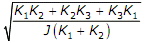# The second moment of a circular area about the diameter is given by (D is the diameter).

1.  πD4/4

2.   πD4/16

3.  πD4/32

4.  πD4/64

4

πD4/64

Explanation :
No Explanation available for this question

# A concentrated load of P acts on a simply supported beam of span L at a distance l/3 from the left support. The bending moment at the point of application of the load is given by

1.  PL/3

2.  2PL/3

3.  PL/9

4.  2PL/9

4

2PL/9

Explanation :
No Explanation available for this question

# Two identical circular rods of same diameter and same length are subjected to same magnitude of axial tensile force. One of the rods is made out of mild steel having the modulus of elasticity of 206 Gpa. The other rod is made out of cast iron having he modulus of elasticity of 100 Gpa. Assume both the materials to be homogeneous and isotropic and the axial force causes the same amount of uniform stress in both the rods. The stresses developed are within the proportional limit of the respective materials. Which of the following observations is correct

1.  As the stresses are equal strains are also equal in both the rodsBoth rods elongate by the same amount

2.  Mild steel rod elongates more than the cast iron rod

3.  Cast iron rod elongates more than the mild steel rod

4.  As the stresses are equal strains are also equal in both the rods

4

Cast iron rod elongates more than the mild steel rod

Explanation :
No Explanation available for this question

# Two beams, one having square cross section and another circular cross-section, are subjected to the same amount of bending moment. If the cross sectional area as well as the material of both the beams are the same then

1.  maximum bending stress developed in both the beams is the same

2.  the circular beam experiences more bending stress that the square one

3.  the square beam experiences more bending stress than the circular one

4.  as the material is same both the beams will experience same deformation

4

the circular beam experiences more bending stress that the square one

Explanation :
No Explanation available for this question

# The mechanism used in a shaping machine is

1.  a closed 4-bar chain having 4 revolute pairs

2.   a closed 6-bar chain having 6 revolute pairs

3.   a closed 4-bar chain having 2 revolute and 2 sliding pairs

4.  an inversion of the single slider-crank chain

4

a closed 4-bar chain having 2 revolute and 2 sliding pairs

Explanation :
No Explanation available for this question

# The lengths of the links of a 4-bar linkage with revolute pairs only are p,q,r and s units.Given that p < q < r < s. which of these links should be the fixed one, for obtaining a ‘double crank’ mechanism

4

Explanation :
No Explanation available for this question

# Consider the arrangement shown in the figure below where J is the combined polar mass moment of inertia of the disc and the shafts. K1, K2, K3 are the torsional stiffness of the respective shafts. The natural frequency of torsional oscillation of the disc is given by

1.

2.

3.

4.

4Explanation :
No Explanation available for this question

# Maximum shear stress developed on the surface of a solid circular shaft under pure torsion is 240 MPa. If the shaft diameter is doubled then the maximum shear stress developed corresponding to the same torque will be

1.  120 mpa

2.   60 mpa

3.  30 mpa

4.   15 mpa

4

30 mpa

Explanation :
No Explanation available for this question

# A wire rope is designated as 6 x 19 standard hoisting. The numbers 6 x 9 represen

1.  diameter in millimeter x length in mete

2.  diameter in centimeter x length in meter

3.  number of strands x number of wires in each strand

4.  number of wires in each strand x number of strands

4

number of strands x number of wires in each strand

Explanation :
No Explanation available for this question

1.  ρghA

2.   (ρs-ρ)ghA

3.   (ρ-ρs)ghA

4.  (ρH-ρsH)ghA

4